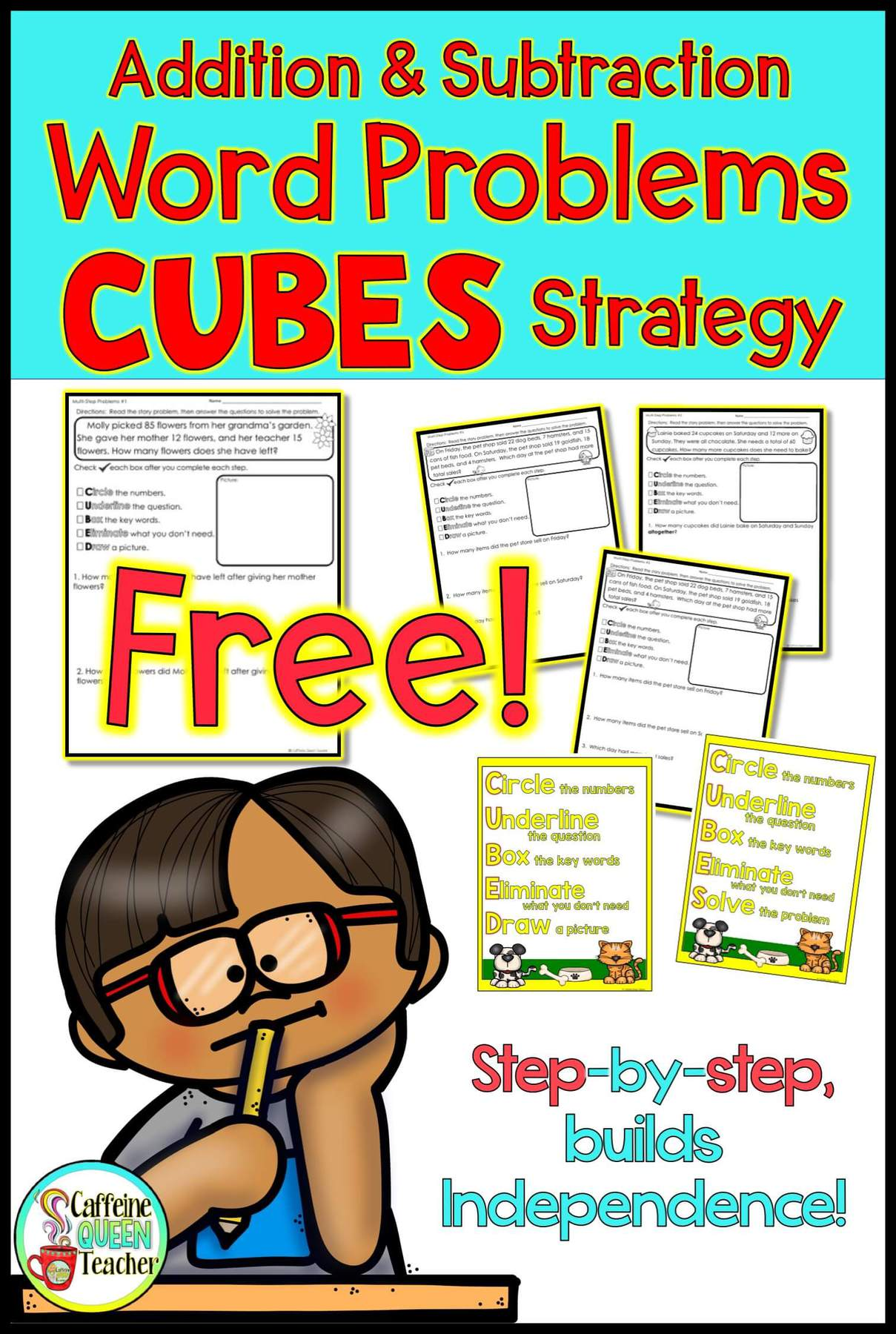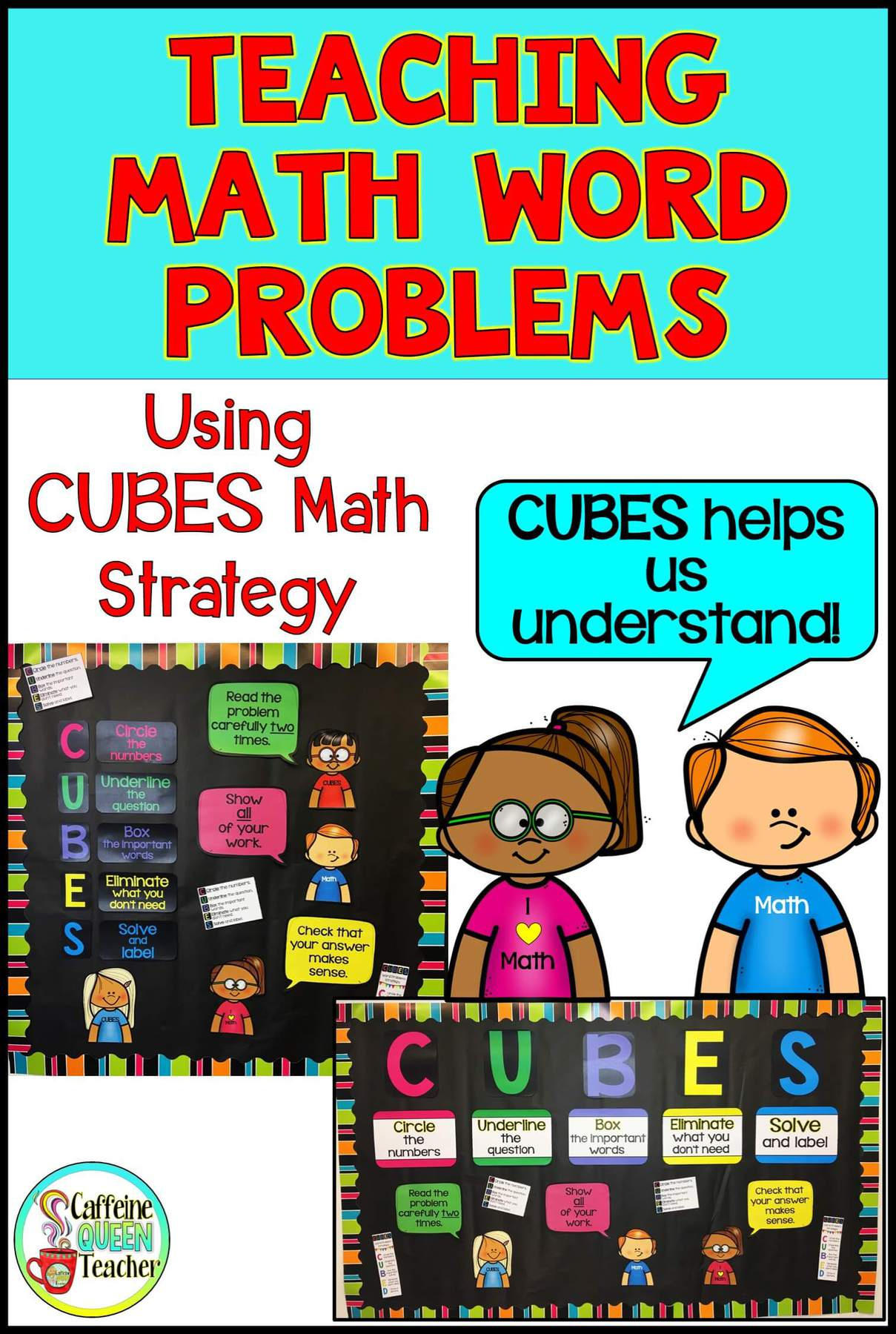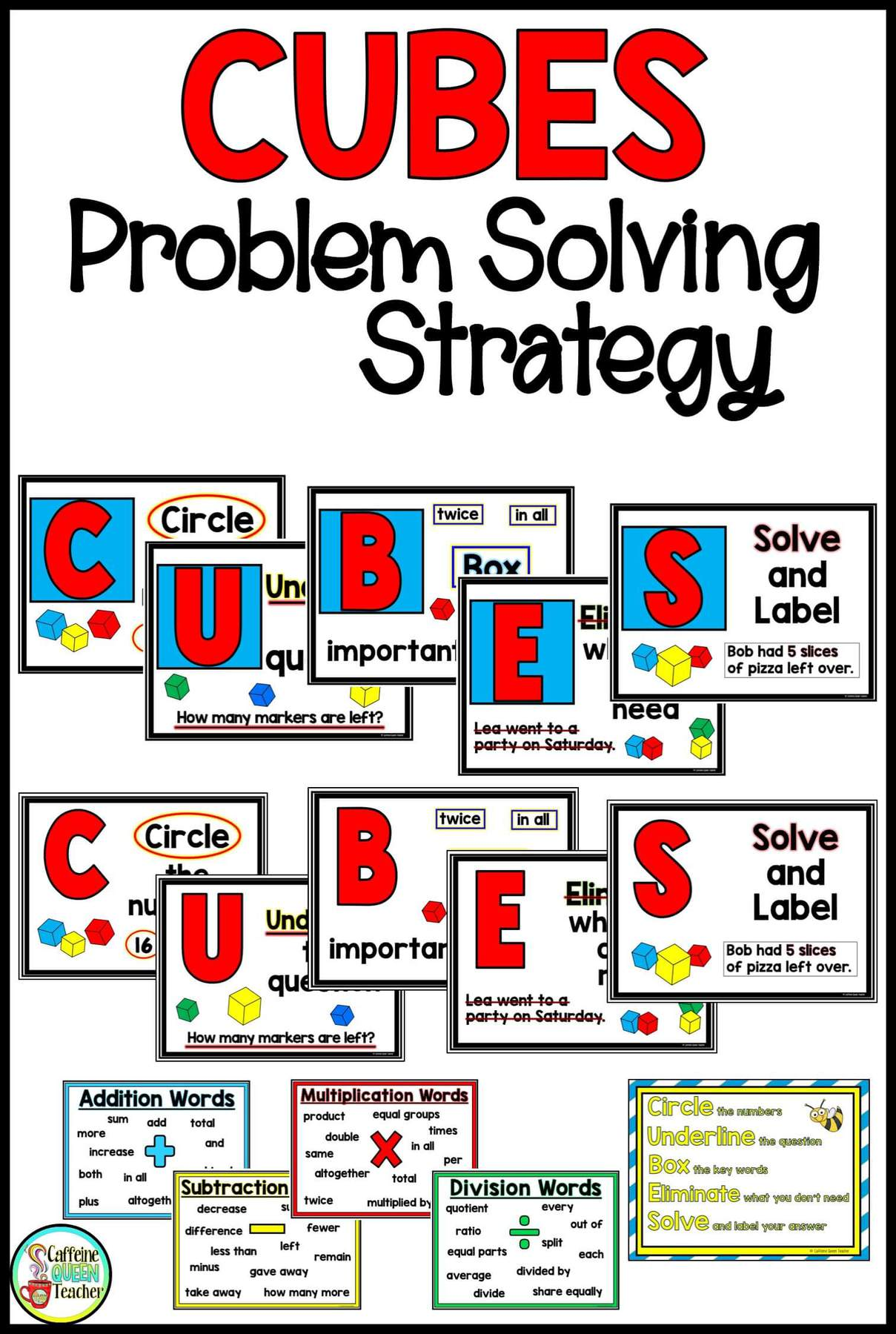# Tag: CUBES### FREE Worksheet – Addition and Subtraction Word Problems Strategy

Free word problem comprehension strategy teaches students to apply reading comprehension strategies using CUBES or CUBED method – FREE!   This easy-to-use CUBES word problem strategy provides your students with the tools to solve addition and subtraction word problems. This strategy works because students are given a solid, concrete method### How to Teach Math Word Problems – CUBES Math Strategy

“Using CUBES math strategy for word problems has been a game-changer!” a teacher from Missouri told me. Having a math problem-solving strategy for story problems is essential. Students need a starting point in dealing with all of those words! Math Word Problems Word problems are here to stay.  They pop